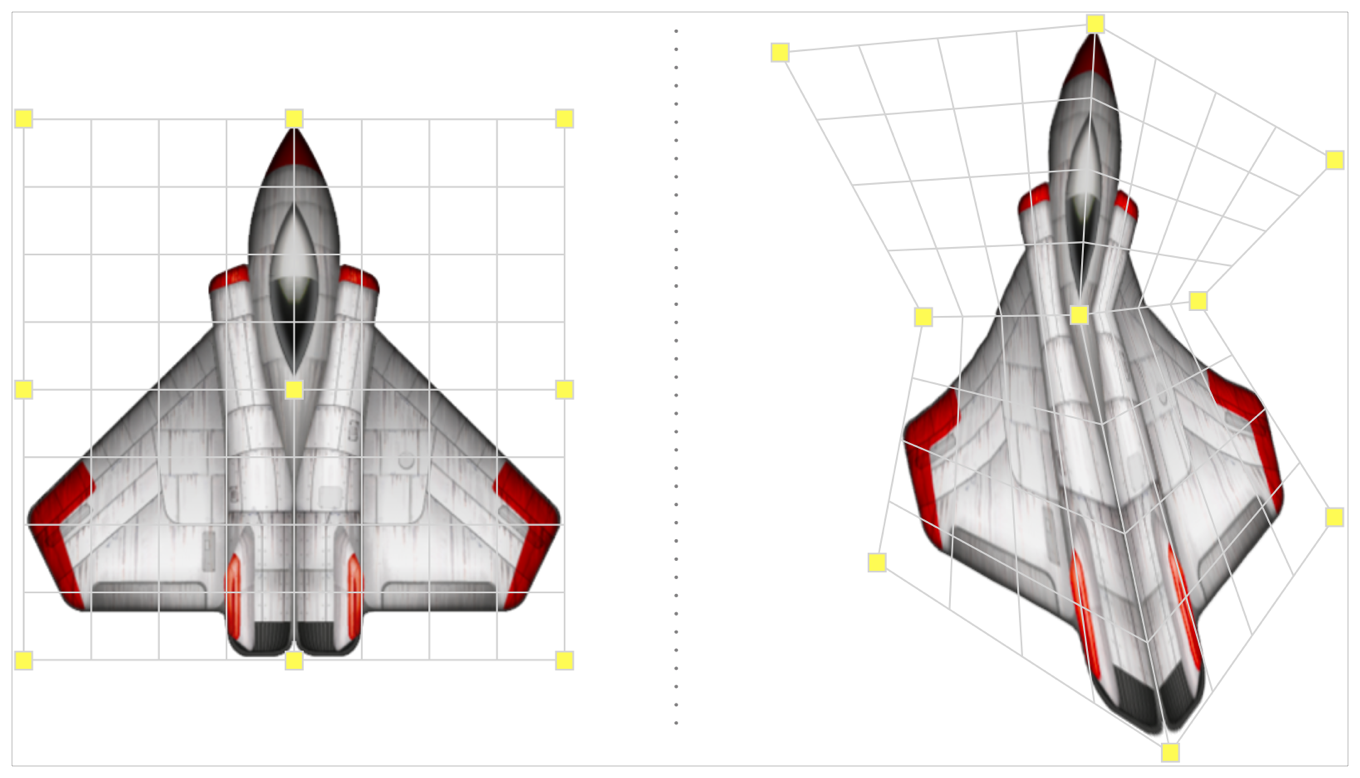# Animate the Warping of a Sprite

Interpolate warping from source to destination warp geometry grids.

### Overview

You create an SKWarpGeometryGrid by supplying two arrays of normalized vertex positions. The sourcePositions array defines the positions of the vertices in the unwarped geometry and the destinationPositions array defines the final, warped destination positions for the source vertices. Both arrays are one dimensional and in row-major order, which means that you additionally have to supply the number of columns and rows of the geometry.

The number of columns or rows is one less than the number of horizontal or vertical vertices, respectively.

The origin of the vertex positions is in the bottom left of the grid. The following code shows how you can create an array of evenly spaced vertices that could act as a default set of source positions for a warp geometry with two columns and two rows. The first item in the array refers to the position at the bottom left, and the last item refers to the position at the top right.

``````let sourcePositions: [float2] = [
float2(0, 1),   float2(0.5, 1),   float2(1, 1),
float2(0, 0.5), float2(0.5, 0.5), float2(1, 0.5),
float2(0, 0),   float2(0.5, 0),   float2(1, 0)
]
``````

If you wanted to give the effect of horizontally squeezing a grid defined by the above vertices around its middle — which would cause it to stretch vertically — you could use a set of destination positions, as shown in the following code:

``````let destinationPositions: [float2] = [
float2(-0.25, 1.5), float2(0.5, 1.75), float2(1.25, 1.5),
float2(0.25, 0.5),   float2(0.5, 0.5),   float2(0.75, 0.5),
float2(-0.25, -0.5),  float2(0.5, -0.75),  float2(1.25, -0.5)
]
``````

You use the sourcePositions and destinationPositions arrays to define a warp geometry object.

``````let warpGeometryGrid = SKWarpGeometryGrid(columns: 2,
rows: 2,
sourcePositions: sourcePositions,
destinationPositions: destinationPositions)
``````

A geometry grid warps the geometry defined by the source positions (left illustration) into a new geometry defined by the destination positions (right illustration).Several options are available for applying this geometry to a node that conforms to `SKWarpable`, such as an `SKSpriteNode`.

The geometry can be applied immediately, without animation, by setting the nodes’s `warpGeometry` property. You may elect to use this technique if you are calculating and applying the destination positions with each scene update; for example, in response to a user’s touch.

A single warp can be applied over time to give an animated morphing effect using the action created by `warp(to:duration:)`. To use a warp action, you first need to define an initial geometry and set it as the `warpGeometry` of the node you want to warp. The `init(columns:rows:)` initializer creates a suitable geometry that has no distortion.

In the following code, the stretched warp geometry created above is used to initialize a warp action with a duration specified in seconds. The node’s run method executes the action.

``````let sprite = SKSpriteNode()
let warpGeometryGridNoWarp = SKWarpGeometryGrid(columns: 2, rows: 2)
sprite.warpGeometry = warpGeometryGridNoWarp
let warpAction = SKAction.warp(to: warpGeometryGrid,duration: 0.5)
sprite.run(warpAction!)
``````

You can chain together multiple warp geometries to create complex morphing animations. For example, you may want to begin with the warp geometry that does not deform the node, morph to the stretched geometry at 0.5”, and finish the sequence by returning to the first geometry at 0.75”. The following code creates this action, using the `animate(withWarps:times:)` method.

``````let warpAction = SKAction.animate(withWarps:[
warpGeometryGridNoWarp,
warpGeometryGrid,
warpGeometryGridNoWarp],
times: [0.25, 0.5, 0.75])
``````

Objects that subclass `SKNode` but don’t conform to `SKWarpable` — for example, `SKShapeNode`, `SKEmitterNode`, or `SKVideoNode` — can be warped by adding them as children of an `SKEffectNode` object. You can, for example, use this approach to distort vector artwork supplied as simple geometric primitives or `CGPath` objects and rendered with a shape node. Warping a particle emitter node that has been added as a child of an effect node allows precise control of the overall shape of a particle system.